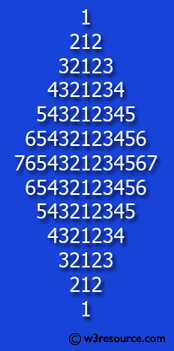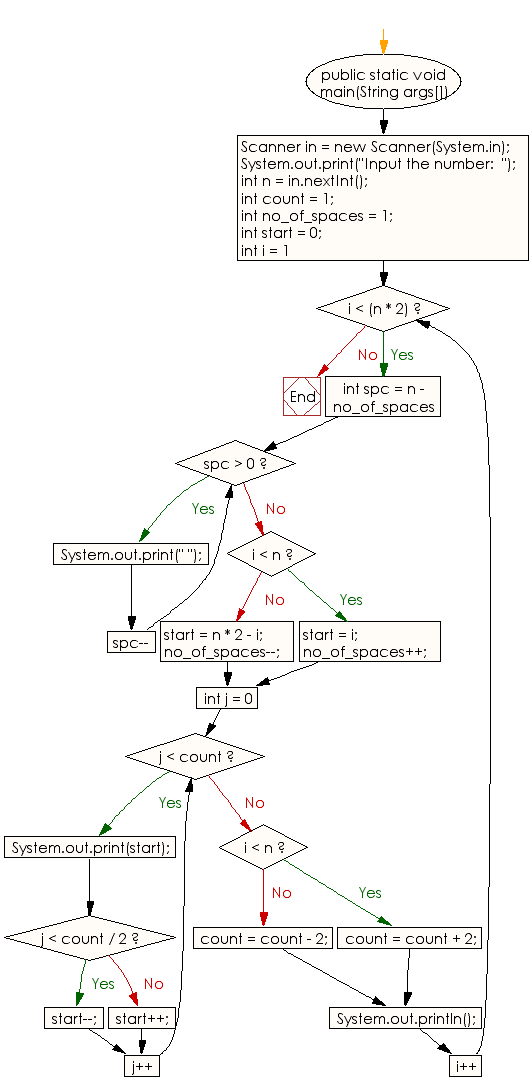﻿ Java exercises: Display the number rhombus structure - w3resource

Sample Solution:# Java Conditional Statement Exercises: Display the number rhombus structure

## Java Conditional Statement: Exercise-25 with Solution

Write a java program to display the number rhombus structure.

Test Data
Input the number: 7

Pictorial Presentation:Sample Solution:

Java Code:

``````import java.util.Scanner;
public class Exercise25 {
public static void main(String args[])
{
Scanner in = new Scanner(System.in);
System.out.print("Input the number:  ");
int n = in.nextInt();
int count = 1;
int no_of_spaces = 1;
int start = 0;

for (int i = 1; i < (n * 2); i++)
{

for (int spc = n - no_of_spaces; spc > 0; spc--)
{
System.out.print(" ");
}
if (i < n)
{
start = i;          //for number
no_of_spaces++;    //for spaces
} else
{
start = n * 2 - i;   //for number
no_of_spaces--;      //for space
}
for (int j = 0; j < count; j++)
{
System.out.print(start);
if (j < count / 2)
{
start--;
} else
{
start++;
}
}
if (i < n)
{
count = count + 2;
} else {
count = count - 2;
}

System.out.println();
}
}
}
```
```

Sample Output:

```Input the number:  7
1
212
32123
4321234
543212345
65432123456
7654321234567
65432123456
543212345
4321234
32123
212
1
```

Flowchart:Java Code Editor: